# Autoencoders and VAEs

Updated:

## 1 Autoencoders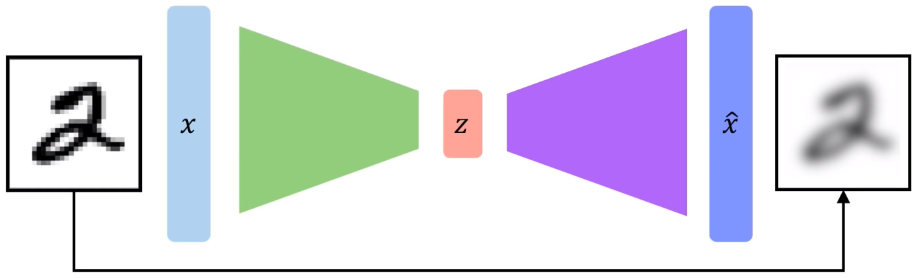The main idea of autoencoders is to extract latent features that are not easily observable yet play an important role in one or several aspects of the data (e.g., images).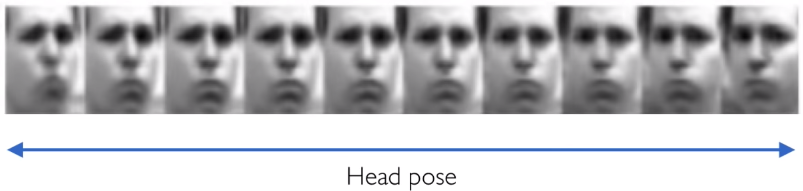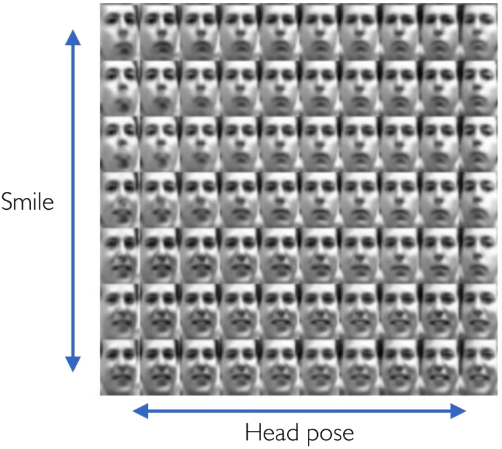Embedding of faces [Saul & Roweis]

### 1.1 Compression (by the “encoder”)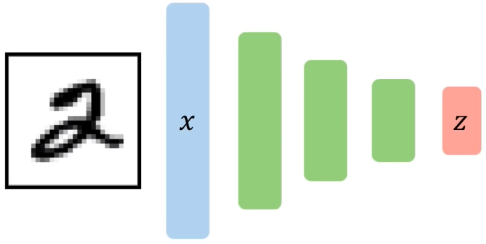The first step of the process is to compress the observed data vector $\vec x$ into the latent feature vector $\vec z$.

There are two obvious benefits yielded from such compression process:

1. The latent feature vector $\vec z$ is much smaller in size, which makes them much easier to process compared to the original (potentially high dimensional) data.
2. As its name suggests, the latent feature vector $\vec z$ may capture important hidden features.

### 1.2 Reconstruction (by the “decoder”)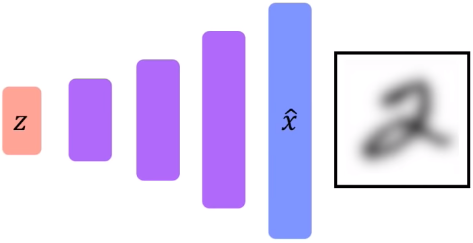The second phase is to try to reproduce the data (the image) from the latent feature vector $\vec z$.

Apparently, since the first step is a “lossy compression”, the data reconstructed $\vec{\hat{x}}$ will not be exactly the same as the original observation. Here is where the third phase comes about.

### 1.3 Backpropagation

As mentioned above, there is a difference between the observation $\vec{x}$ and the reconstruction $\vec{\hat{x}}$.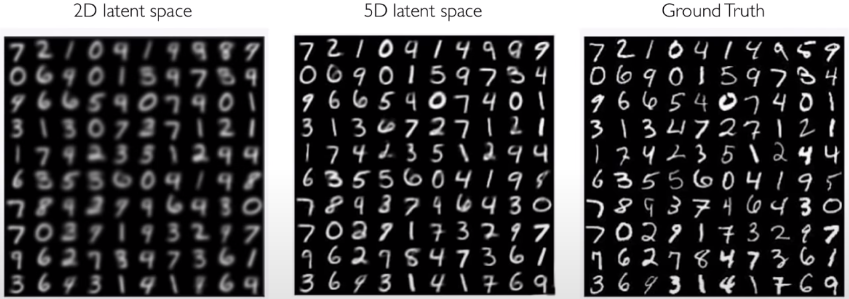From the above picture, we can see clearly that the higher the dimension of the latent feature vector $\vec{z}$, the higher the quality of the reconstruction.

Therefore, constraining the size of the latent space will enforce the “importance” of the extracted features.

Further, we can use a loss function to measure such “importance” of the extracted hidden variables. In this case, we use a simple square loss:

$\mathcal{L}(x, \hat{x})=\|x-\hat{x}\|^{2}$

Thus, the key power of autoencoders is that

Autoencoder allows us to quantify the latent variables without labels (gold-standard data)!

To summarize,

• Autoencoding == Automatically encoding data
• Bottleneck hidden layer forces the network to learn a compressed latent representation.
• Reconstruction loss forces the latent representation be as “paramount” and “informative” as possible.

## 2 Variational Autoencoders (VAEs)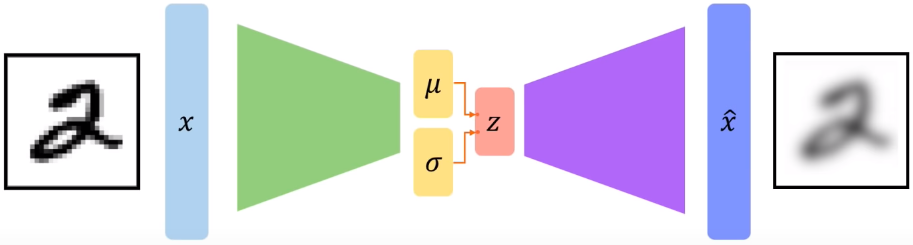### 2.1 Stochastical variation

In a nutshell, variational autoencoders are a probabilistic twist on autoencoders, i.e. (stochastically) sample from the mean and standard deviation to compute the latent sample as supposed to deterministically take the entire latent vector $\vec{z}$. That been said, the main idea of the forward propagation does not change compared to traditional autoencoders.

• In the compression process, the encoder computes $p_{\phi}(\mathrm{z} \mid x)$.
• In the reconstruction phase, the decoder computes $q_{\theta}(\mathrm{x} \mid z)$.

Then, we could compute the loss as follows

$\mathcal{L}(\phi, \theta, x)=(\text { reconstruction loss })+(\text { regularization term }),$

which is exactly the same as before. It captures the pixel-wise difference between the input and the reconstructed output. This is a metrics of how well the network is doing at generating the distribution that akin to that of the observation.

As to the “regularization term”, since the VAE is producing these probability distributions, we want to place some constraints on how they are computed as well as what that probability distribution resembles as a part of regularizing and training the network.

Hence, we place a prior $p(z)$ on the latent distribution as follows

$D(p_{\phi}(z|x)\ ||\ p(z)),$

which captures the KL divergence between the inferred latent distribution and this fixed prior for which a common choice is a normal Gaussian, i.e. we centre it around with a mean of 0 and a standard deviation 1: $\ p(z)=\mathcal{N}\left(\mu=0, \sigma^{2}=1\right)$.

In this way, the network will learn to penalise itself when it tries to cheat and cluster points outside sort of this smooth Gaussian distribution as it would be the case if it was overfitting or trying to memorize particular instances of the input.

Thus, this will enforce the extracted $\vec z$ follows the shape of our initial hypothesis about the distribution, smoothing out the latent space and, in turn, helping the network not over-fit on certain parts of the latent space.

### 2.2 Backpropagation? Reparametrization

Unfortunately, due to the stochastic nature, the backpropagation cannot pass the sampling layer as backpropagation requires deterministic nodes to be able to iteratively pass gradients and apply the chain rule through.

Instead, we consider the sampled latent vector $\vec z$ as a sum of a fixed vector $\vec \mu$ a fixed variance vector $\vec \sigma$ and then scaled this variance vector by a random constant that is drawn from a prior distribution, for example from a normal Gaussian. The key idea here is that we still have a stochastic node but since we have done this reparametrization with the factor $\epsilon$ that is drawn from a normal distribution, this stochastic sampling does not occur directly in the bottleneck layer of $\vec z$. This way, we can reparametrize where that sampling is occurring.

Note that this is a really powerful trick as such reparametrization is what allows for VAEs to be trained end-to-end.

## 3 Code example

The following is a vanila implementation of a VAE model in Tensorflow.

class Sampling(keras.layers.Layer):
def call(self, inputs) :
z_mean, z_log_var = inputs
batch = tf.shape(z_mean)
dim = tf.shape(z_mean)
epsilon = tf.keras.backend.random_normal_(shape=(batch, dim))
return z_mean + tf.exp(0.5 * z_log_var)

latenet_dim = 2
encoder_inputs = Input(shape=(6), name="input_layer")

X = Dense (5, activation="relu", name="h1")(encoder_inputs)
X = Dense (5, activation="relu", name="h2")(x)
X = Dense (4, activation="relu", name="h3")(x)
z_mean = Dense(latent_dim, name="z_mean")(x)
z_log_var = Dense(latent_dim, name="z_log_var")(x)
z = Sampling()([z_mean, z_log_var])

encoder = keras.Model(encoder_inputs, [z_mean, z_log_var, z], name="encoder")

keras.utils.plot_model(encoder, show_shapes=True)


Tags: